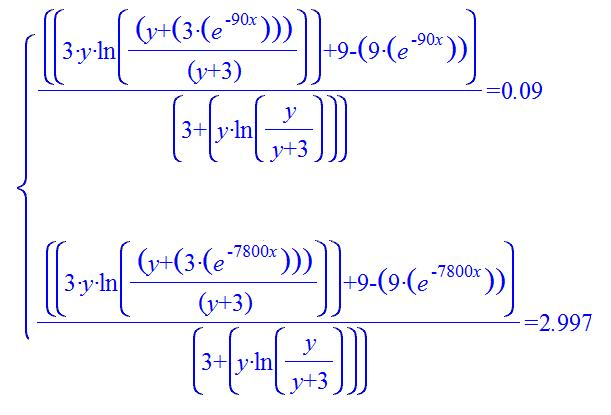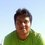# There are real solutions?Can $y$ and $x$ be real numbers? Does this system have solutions? Chemical reaction S+E=SE=P+ENote by Raffaele Piccirillo
5 years, 4 months ago

This discussion board is a place to discuss our Daily Challenges and the math and science related to those challenges. Explanations are more than just a solution — they should explain the steps and thinking strategies that you used to obtain the solution. Comments should further the discussion of math and science.

When posting on Brilliant:

• Use the emojis to react to an explanation, whether you're congratulating a job well done , or just really confused .
• Ask specific questions about the challenge or the steps in somebody's explanation. Well-posed questions can add a lot to the discussion, but posting "I don't understand!" doesn't help anyone.
• Try to contribute something new to the discussion, whether it is an extension, generalization or other idea related to the challenge.

MarkdownAppears as
*italics* or _italics_ italics
**bold** or __bold__ bold
- bulleted- list
• bulleted
• list
1. numbered2. list
1. numbered
2. list
Note: you must add a full line of space before and after lists for them to show up correctly
paragraph 1paragraph 2

paragraph 1

paragraph 2

[example link](https://brilliant.org)example link
> This is a quote
This is a quote
    # I indented these lines
# 4 spaces, and now they show
# up as a code block.

print "hello world"
# I indented these lines
# 4 spaces, and now they show
# up as a code block.

print "hello world"
MathAppears as
Remember to wrap math in $$ ... $$ or $ ... $ to ensure proper formatting.
2 \times 3 $2 \times 3$
2^{34} $2^{34}$
a_{i-1} $a_{i-1}$
\frac{2}{3} $\frac{2}{3}$
\sqrt{2} $\sqrt{2}$
\sum_{i=1}^3 $\sum_{i=1}^3$
\sin \theta $\sin \theta$
\boxed{123} $\boxed{123}$

Sort by:

Woah. What were you trying to solve?

- 5 years, 4 months ago

Modelling an enzimatic reaction :)

- 5 years, 4 months ago

Using? A variation of Michelis-Menten?

- 5 years, 4 months ago

Yes =) stating the rate of conversion x in different way :)

- 5 years, 4 months ago

Haha woah cool! I knew it would be that :P
What about approximations? If you assume some concentrations/rate constants are much larger than others, then you can neglect many terms. But I'm sure you've thought about this already.

- 5 years, 4 months ago

this is already the reducted model :) but the two therms can't be calculated so easy by my pc xD

- 5 years, 4 months ago

Are x and y concentrations?
If so, then there must be some real solution, right? (as we're modelling a real-world chemical reaction.)

- 5 years, 4 months ago

0<y<1 and 0<x<0.1; y is a constant;x is the inverse of a time =)

- 5 years, 4 months ago

Ah okay, now I'm seeing the problem. I can't really help you much more though - have you tried Mathematica Online?

- 5 years, 4 months ago

Not yet,I don't know the website :)

- 5 years, 4 months ago

Oh sure, here it is. There's a fifteen day free trial, which should be enough for your computations.

- 5 years, 4 months ago

Thank you =)

- 5 years, 4 months ago